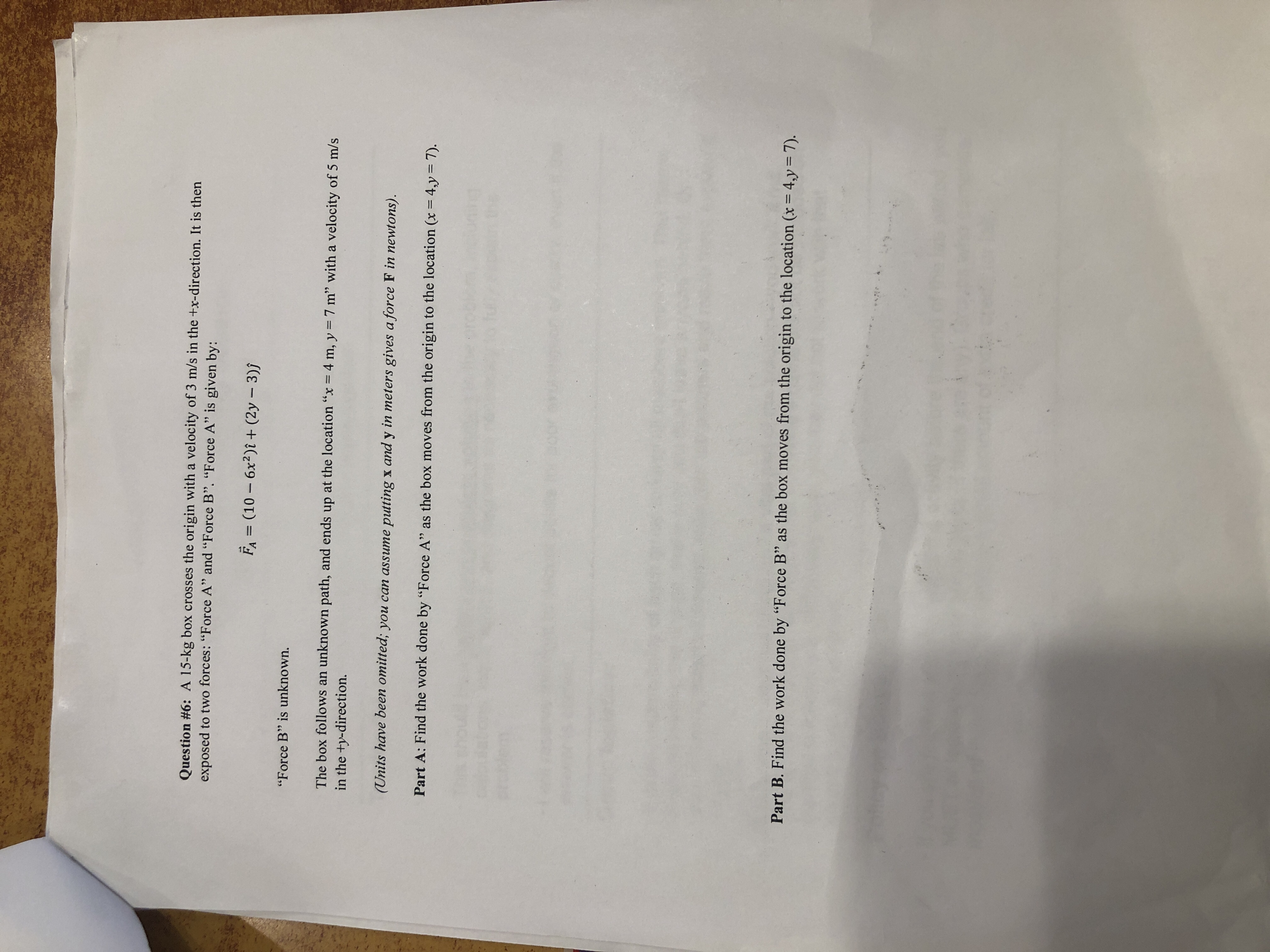Question #6: A 15-kg box crosses the origin with a velocity of 3 m/s in the +x-direction. It is thenexposed to two forces. "Force A" and "Force B". "Force A" is given by:F, (10 -6x2)(2y-3))"Force B" is unknown.The box follows an unknown path, and ends up at the location "x4 m, y7 m" with a velocity of 5 m/sin the ty-direction.(Units have been omitted; you can assume putting x and y in meters gives a force FiPart A:Find the work done by "Force A'" as the box moves from the origin to the location (x 4,y 7)Part B. Find the work done by "Force B" as the box moves from the origin to the location (x4,y7)

Question

Hw review please show work formula question 6help_outlineImage TranscriptioncloseQuestion #6: A 15-kg box crosses the origin with a velocity of 3 m/s in the +x-direction. It is then exposed to two forces. "Force A" and "Force B". "Force A" is given by: F, (10 -6x2)(2y-3)) "Force B" is unknown. The box follow s an unknown path, and ends up at the location "x 4 m, y 7 m" with a velocity of 5 m/s in the ty-direction. (Units have been omitted; you can assume putting x and y in meters gives a force Fi Part A: Find the work done by "Force A'" as the box moves from the origin to the location (x 4,y 7) Part B. Find the work done by "Force B" as the box moves from the origin to the location (x 4,y 7) fullscreen
Step 1

(A) Work is done whenever a force moves something over a distance. Work done is the intergral of force and displacement  which is given in equation (1).

Step 2

(B)

According to work energy theorem, the work done by the sum of all forces acting...

Want to see the full answer?

See Solution

Want to see this answer and more?

Our solutions are written by experts, many with advanced degrees, and available 24/7

See Solution
Tagged in

Work,Power and Energy